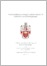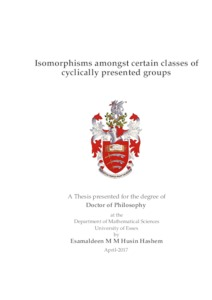# Isomorphisms amongst certain classes of cyclically presented groups

Hashem, Esamaldeen M M Husin (2017) Isomorphisms amongst certain classes of cyclically presented groups. PhD thesis, University of Essex.Preview
Text
thesis.pdf

Download (562kB) | Preview

## Abstract

In this thesis we consider isomorphisms amongst certain classes of cyclically presented groups. We give isomorphism theorems for two families of cyclically presented groups, the groups G_n(h, k, p, q,r,s, l), and the groups G^ε_n(m, k, h), which were introduced by Cavicchioli, Repovs and Spaggiari. These families contain many subfamilies of cyclically presented groups, we have results for two of them, the groups G_n(m, k), which were introduced by Johnson and Mawdesley, and the groups Γ_n(k, l), which were introduced by Cavicchioli, Repovs and Spaggiari. The abelianization of the Fibonacci groups F(2, n) was proved by Lyndon to be finite and its order can be expressed in terms of the Lucas numbers. Bardakov and Vesnin have asked if there is a formula for the order of the abelianization of G_n(m, k) groups that can be expressed in terms of Fibonacci numbers. We produce formulas that compute the order of G_{pm}(x_0x_mx^{±1}_k)^{ab} , G_{pk}(x_0x_mx^{±1}_k)^ab for certain values of p where m, k are coprime, and for the groups Γ_n(1,n/2− 1)^{ab} (this formula is given in terms of Lucas numbers). The values of the number of non-isomorphic G_n(m, k) groups was conjectured by Cavicchioli, O’Brien and Spaggiari for n = p^l, where p is prime and l is a positive integer, we show that these values provide an upper bound for the number of non-isomorphic G_n(m, k) groups. We also give lower bounds and upper bounds for the number of non- isomorphic G_n(m, k) and Γ_n(k, l) groups for certain values of n. Similar to the investigation of the type of isomorphisms of G_n(m, k) groups for n ≤ 27 that was carried by Cavicchioli, O’Brien and Spaggiari, we perform a similar investigation for Γn(k, l) groups for n ≤ 29.

Item Type: Thesis (PhD) Q Science > QA Mathematics Faculty of Science and Health > Mathematical Sciences, Department of Esamaldeen Hashem 01 Aug 2017 13:17 01 Aug 2017 13:17 http://repository.essex.ac.uk/id/eprint/20135

### Actions (login required)View Item